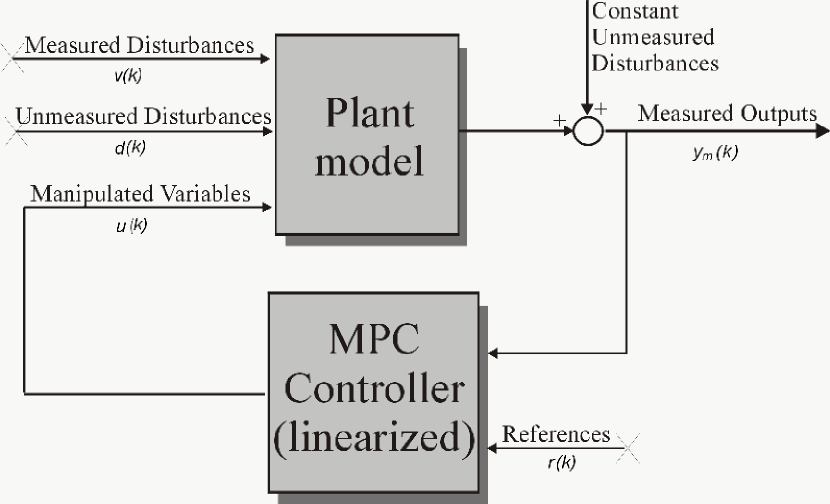# cloffset

Compute closed-loop DC gain from output disturbances to measured outputs assuming constraints are inactive at steady state

## Syntax

``dcgain = cloffset(mpcobj)``

## Description

Use this function to calculate the steady state output sensitivity of the closed loop. A zero value means that the measured plant output can track the desired output reference setpoint.

example

````dcgain = cloffset(mpcobj)` returns the DC gain matrix `dcgain`. `mpcobj` is the MPC object specifying the controller for which the closed-loop gain is calculated.Computing the Effect of Output DisturbancesRelying on the superposition of effects principle, the gain is computed by zeroing references, measured disturbances, and unmeasured input disturbances.```

## Examples

collapse all

Create a plant, a corresponding MPC object, and calculate the closed loop static gain (this is also referred to as steady state loop output sensitivity).

```mpcverbosity off; % turn off mpc messaging plant=tf(1,[1 1],0.2); % create plant (0.2 seconds sampling time) mpcobj=mpc(plant,0.2); % create mpc object (0.2 second sampling time) cloffset(mpcobj) % calculate steady state output sensitivity of closed loop ans = 0 A zero gain (which is typically the result of the controller having % integrators as input or output disturbance models) means that the % measured plant output will track the desired output reference setpoint. zpk(mpcobj) % convert unconstrained MPC to zero/pole/gain form ans = From input "MO1" to output "MV1": 0.45205 z^2 (z-1.5) ----------------------------- (z-1) (z-0.02575) (z+0.02485) Sample time: 0.2 seconds Discrete-time zero/pole/gain model. ```

Converting the unconstrained controller to `zpk` form shows that the pole in `z=1`, (resulting from the default noise model being an integrator), causes the controller static gain to approach infinity, in turn causing the closes loop output sensitivity to be zero at steady state (`z=1`). This allows the controller to successfully track the output reference signal.

## Input Arguments

collapse all

Model predictive controller, specified as an MPC controller object. To create an MPC controller, use `mpc`.

## Output Arguments

collapse all

The steady state closed loop output sensitivity `dcgain` is an nym-by-nym matrix , where nym is the number of measured plant outputs.`dcgain(i,j)` represents the gain from an additive (constant) disturbance on output `j` to measured output `i`. If row `i` contains all zeros, there will be no steady-state offset on output `i`, and that the controller can achieve perfect tracking of the `i`th component of an output reference setpoint (assuming constraints are inactive at steady state).

## Version History

Introduced before R2006a